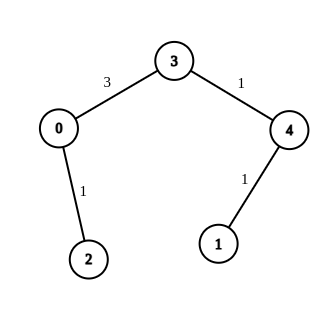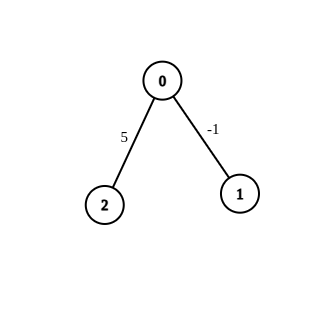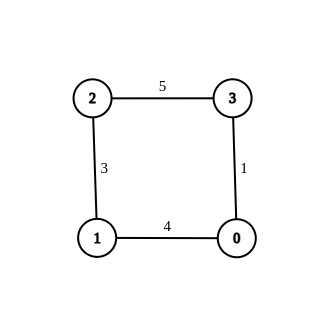2699. Modify Graph Edge Weights
Hard
165
31

You are given an undirected weighted connected graph containing `n` nodes labeled from `0` to `n - 1`, and an integer array `edges` where `edges[i] = [ai, bi, wi]` indicates that there is an edge between nodes `ai` and `bi` with weight `wi`.

Some edges have a weight of `-1` (`wi = -1`), while others have a positive weight (`wi > 0`).

Your task is to modify all edges with a weight of `-1` by assigning them positive integer values in the range `[1, 2 * 109]` so that the shortest distance between the nodes `source` and `destination` becomes equal to an integer `target`. If there are multiple modifications that make the shortest distance between `source` and `destination` equal to `target`, any of them will be considered correct.

Return an array containing all edges (even unmodified ones) in any order if it is possible to make the shortest distance from `source` to `destination` equal to `target`, or an empty array if it's impossible.

Note: You are not allowed to modify the weights of edges with initial positive weights.

Example 1:```Input: n = 5, edges = [[4,1,-1],[2,0,-1],[0,3,-1],[4,3,-1]], source = 0, destination = 1, target = 5
Output: [[4,1,1],[2,0,1],[0,3,3],[4,3,1]]
Explanation: The graph above shows a possible modification to the edges, making the distance from 0 to 1 equal to 5.
```

Example 2:```Input: n = 3, edges = [[0,1,-1],[0,2,5]], source = 0, destination = 2, target = 6
Output: []
Explanation: The graph above contains the initial edges. It is not possible to make the distance from 0 to 2 equal to 6 by modifying the edge with weight -1. So, an empty array is returned.
```

Example 3:```Input: n = 4, edges = [[1,0,4],[1,2,3],[2,3,5],[0,3,-1]], source = 0, destination = 2, target = 6
Output: [[1,0,4],[1,2,3],[2,3,5],[0,3,1]]
Explanation: The graph above shows a modified graph having the shortest distance from 0 to 2 as 6.
```

Constraints:

• `1 <= n <= 100`
• `1 <= edges.length <= n * (n - 1) / 2`
• `edges[i].length == 3`
• `0 <= ai, bi < n`
• `wi = -1 `or `1 <= wi <= 107`
• `ai != bi`
• `0 <= source, destination < n`
• `source != destination`
• `1 <= target <= 109`
• The graph is connected, and there are no self-loops or repeated edges
Accepted
2.3K
Submissions
11.7K
Acceptance Rate
19.3%

Seen this question in a real interview before?
1/4
Yes
No

Discussion (0)

Related Topics